# Quantum mechanics - infinite square well problem

• Graham87
In summary, the conversation revolved around solving the integral in part d) of a problem. The person had successfully solved part c) but was struggling with the integral in part d). They considered rewriting part c) into an initial wave function with constants, but were unsure how to proceed. Eventually, they were able to relate the integral to one of the eigenfunctions and use orthonormality to solve it. It was suggested to use general properties of eigenfunctions as much as possible.f

#### Graham87

Homework Statement
For a wave function evaluate the following integral
Relevant Equations
Infinite square well equations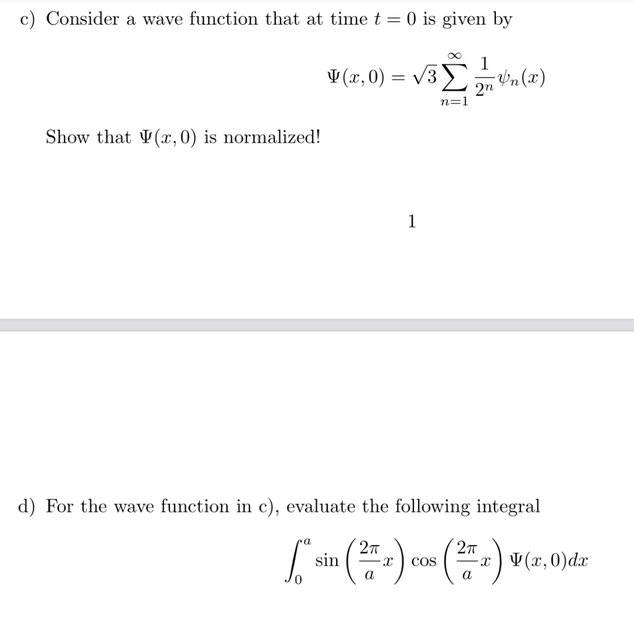I have solved c), but don’t know how to solve the integral in d.
It looks like an integral to get c_n (photo below), but I still can’t figure out what to make of c) in the integral of d).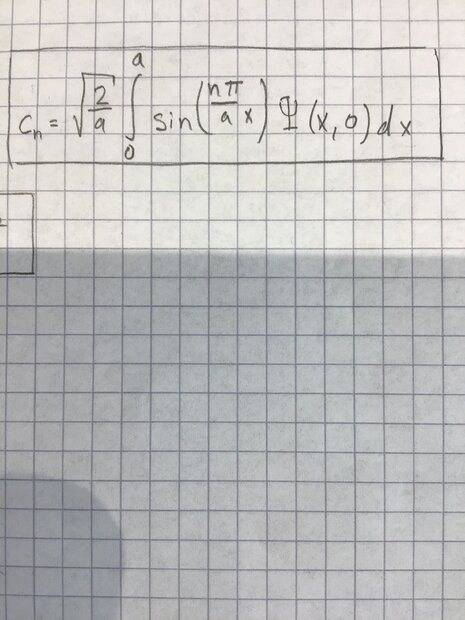I also thought maybe you can rewrite c) into an initial wave function (photo below) with A,x,a but don’t know how.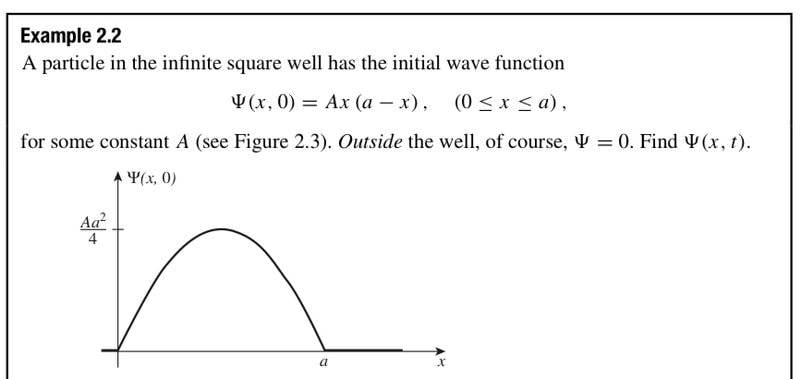Thanks!

•Delta2
I have solved c), but don’t know how to solve the integral in d.

Consider using a trig identity to write ##\sin\left(\frac{2\pi}{a}x\right)\cos\left(\frac{2\pi}{a}x\right)## in a way that will be helpful in evaluating the integral.

•Graham87 and Delta2
I tried like this already, but I still don’t know how to deal with c) in the integral.
OK, good. Can you relate ##\large\frac{\sin\left(\frac{4\pi x}{a}\right)}{2}## to one of the ##\psi_n(x)##?

•Graham87 and Delta2
OK, good. Can you relate ##\large\frac{\sin\left(\frac{4\pi x}{a}\right)}{2}## to one of the ##\psi_n(x)##?
ψ4(x) ?

•Delta2
ψ4(x) ?
Exactly what is the relation between ##\large\frac{\sin\left(\frac{4\pi x}{a}\right)}{2}## and ##\psi_4(x)##?

•Graham87 and Delta2
Exactly what is the relation between ##\large\frac{\sin\left(\frac{4\pi x}{a}\right)}{2}## and ##\psi_4(x)##?
Same n?
How does this look?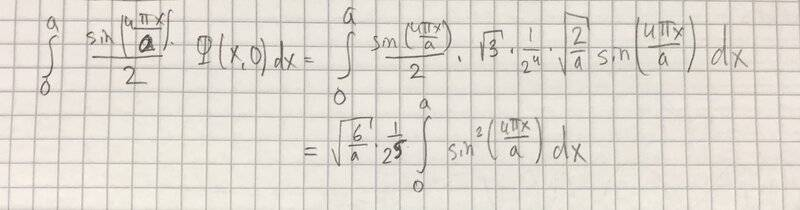I don’t think it’s correct because c_4 is not the same answer.

How does this look?View attachment 303556
That will work.

However, it's maybe a little nicer to show ##\large\frac{\sin\left(\frac{4\pi x}{a}\right)}{2} = \frac{\sqrt{2a}}{4}\psi_4(x)## . Then you don't need to do any explicit integration. Just use the orthonormality of the ##\psi_n(x)##.

•Delta2, Graham87, PeroK and 1 other person
That will work.

However, it's maybe a little nicer to show ##\large\frac{\sin\left(\frac{4\pi x}{a}\right)}{2} = \frac{\sqrt{2a}}{4}\psi_4(x)## . Then you don't need to do any explicit integration. Just use the orthonormality of the ##\psi_n(x)##.
So like this?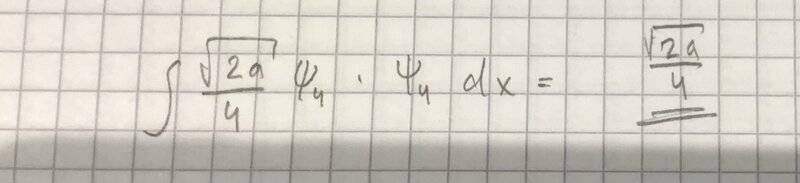I found the integral d) similar to this.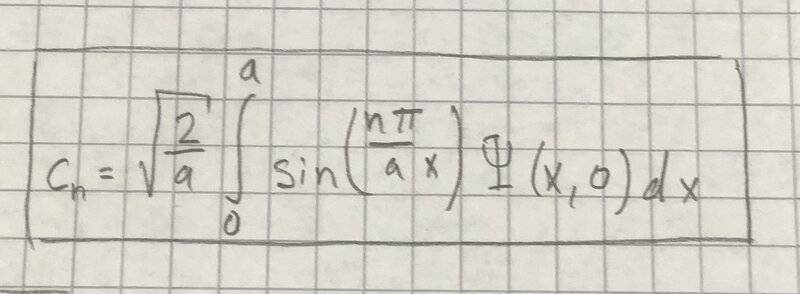Is it wrong to assume that c_4 should be the same as my answer in d)?
In that case my answer in d is not correct.

$$\frac{\sqrt{2a}}{4} \int \psi_4^2\,dx$$ and $$\int \psi_4^2\,dx$$ equal? I think you should be able to answer that on your own. :)

•Graham87
You need to calculate ##\int_0^a{\sin\left(\frac{2\pi x}{a}\right)\cos\left(\frac{2\pi x}{a}\right)}\Psi(x,0)dx##

Write this as ##\frac{\sqrt{2a}}{4}\int_0^a\psi_4(x)\Psi(x,0)dx##.

Substitute the given expression for ##\Psi(x,0)## and evaluate using the orthonormality of the ##\psi_n(x)##.

You should get the same answer as you got in post #7.

••Delta2, PeroK and Graham87
Big thanks! Got it!

•TSny
Big thanks! Got it!
In general, it's a good habit to use the notation ##\psi_n(x)## and use the general properties of eigenfunctions - especially orthonormality - as much as possible. And only resort to the specific eigenfunctions when necessary.

••Graham87, Delta2 and TSny